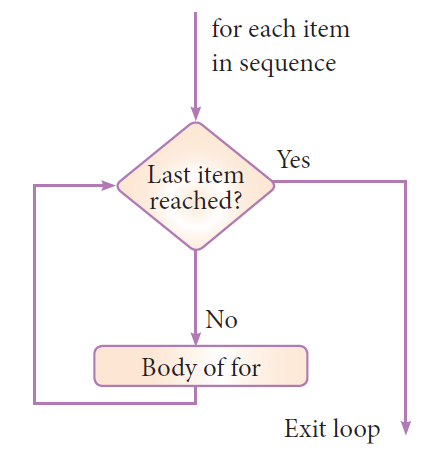#### For loop

for loop is the most comfortable loop. It is also an entry check loop. The condition is checked in the beginning and the body of the loop(statements-block 1) is executed if it is only True otherwise the loop is not executed.

Syntax:

for counter_variable in sequence:

statements-block 1

[else: # optional block

statements-block 2]

The counter_variable mentioned in the syntax is similar to the control variable that we used in the for loop of C++ and the sequence refers to the initial, final and increment value. Usually in Python, for loop uses the range() function in the sequence to specify the initial, final and increment values. range() generates a list of values starting from start till stop-1.Example : #program to illustrate the use of for loop - to print single digit even number

for i in range (2,10,2):

print (i, end=' ')

Output:

2 4 6 8

Example  : #program to illustrate the use of for loop - to print single digit even number with else part

`for i in range(2,10,2):print (i,end=' ')else:print ("\nEnd of the loop")`

Output:

2 4 6 8

End of the loop

Example : # program to calculate the sum of numbers 1 to 100

`n = 100sum = 0for counter in range(1,n+1):	sum = sum + counterprint("Sum of 1 until %d: %d" % (n,sum))`

Output:

Sum of 1 until 100: 5050

Example : program to illustrate the use of string in range() of for loop

`for word in 'Computer':print (word,end=' ')else:print ("\nEnd of the loop")`

Output

C o m p u t e r

End of the loop